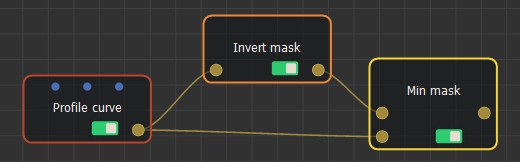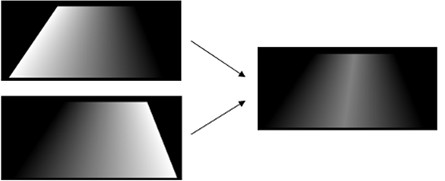### Adding a Min mask node

This node calculates the minimum of two masks. At each vertex, the value of the output mask is the minimum of the values of both input masks.

To add a Min Mask node, right-click in the Graph Editor and select Create Node  > Mask Composition  > Min Mask.This node has no properties.

### Linking to input masks

Link the Min mask node to two input nodes.The Min Mask node calculates the minimum of the two nodes.

For example:If the input masks do not have the same size or resolution, the size and resolution of the output mask is determined by the size and resolution of the first mask, and the second mask is enlarged or decreased so that its size corresponds exactly to the size of the output mask, before being compared to the first mask.

Copyright © 2021 · All Rights Reserved · Wysilab